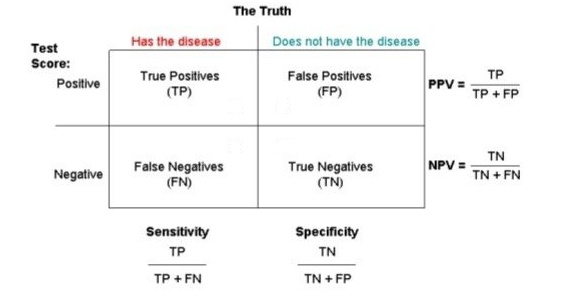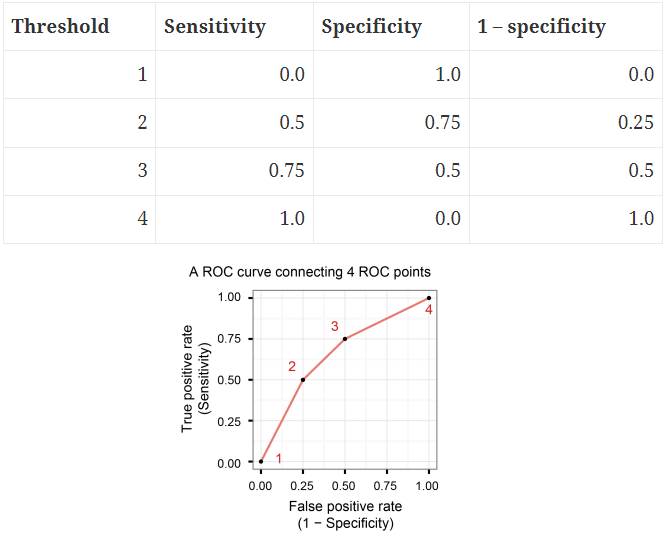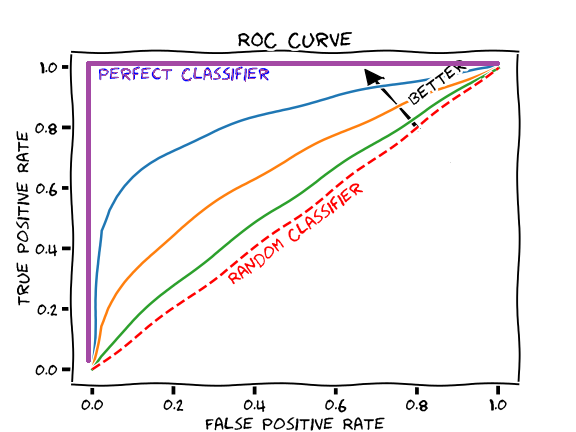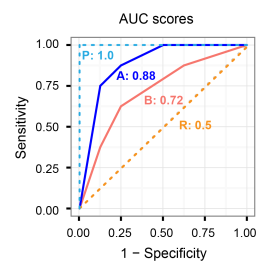# 1 Classification. Sensitivity and specificity. Area under the ROC curve

We may use the logistic regression model as a technique for classification. We would like our model to have high discrimination ability. This means that observations with Y=1 ought to be predicted high probabilities, and those with Y=0 ought to be assigned low probabilities.

## 1.1 Sensitivity and specificitySensitivity (True Positive rate) measures the proportion of positives that are correctly identified (i.e. the proportion of those who have some condition (affected, Y=1) who are correctly identified as having the condition).

Specificity (True Negative rate) measures the proportion of negatives that are correctly identified (i.e. the proportion of those who do not have the condition (unaffected, Y=0) who are correctly identified as not having the condition).

Sensitivity = TP/(TP + FN) = (Number of true positive assessment)/(Number of all positive assessment)

Specificity = TN/(TN + FP) = (Number of true negative assessment)/(Number of all negative assessment)

1-specificity called False Positive Rate

PPV: positive predictive value (or precision) = proportion of those with a POSITIVE test that have the condition

NPV: negative predictive value = proportion of those with a NEGATIVE test that do not have the condition

Accuracy = (TN + TP)/(TN+TP+FN+FP) = (Number of correct assessments)/Number of all assessments)

Our model is perfect at classifying observations if it has 100% sensitivity and 100% specificity. Unfortunately in practice this is (usually) not attainable.

We want our model to have high sensitivity and low values of 1-specificity.

## 1.2 Exercise

For our data set picking a threshold of 0.75 gives us the following results:

FN = 340, TP = 27

TN = 3545, FP = 9

What are the sensitivity and specificity for this particular decision rule?

Sensitivity = TP/(TP + FN) = 27/(27 + 340) = 0.073

Specificity = TN/(FP + TN) = 3545/(9 + 3545) = 0.997

## 1.4 Classification rule

We can create classification rule by choosing a threshold value $$c$$, and classify those observations with a fitted probability above $$c$$ as positive and those at or below it as negative.

For a particular threshold value $$c$$, we can estimate the sensitivity by the proportion of observations with Y=1 which have a predicted probability above $$c$$, and similarly we can estimate specificity by the proportion of Y=0 observations with a predicted probability at or below $$c$$.

If we increase the threshold value $$c$$, fewer observations will be predicted as positive. This will mean that fewer of the Y=1 observations will be predicted as positive (reduced sensitivity), but more of the Y=0 observations will be predicted as negative (increased specificity). In picking the threshold value, there is thus an intrinsic trade off between sensitivity and specificity.

## 1.5 The receiver operating characteristic (ROC) curve

The ROC curve, is a plot of the values of sensitivity (y-axis) against 1-specificity (x-axis), evaluated at values of the threshold value $$c$$ from 0 to 1.A model with high discrimination ability will have high sensitivity and specificity, leading to an ROC curve which goes close to the top left corner of the plot. A model with no discrimination ability will have an ROC curve which is the 45 degree diagonal line.## 1.6 Area under the ROC curve

The higher the AUC, the better the performance of the model at distinguishing between the positive and negative classes.

A way of summarizing the discrimination ability of a model is to report the area under the ROC curve. The area under the curve ranges from 1, corresponding to perfect discrimination (ROC curve to the top left hand corner), to 0.5, corresponding to a model with no discrimination ability (ROC curve which is the 45 degree diagonal line).# 2 Example

The data aSAH summarizes several clinical and one laboratory variable of 113 patients with an aneurysmal subarachnoid hemorrhage

library(pROC)
data(aSAH)
head(aSAH)
res <- glm(outcome ~ gender + age + s100b + ndka, family  = binomial(link = "logit"), data = aSAH)
summary(res)
##
## Call:
## glm(formula = outcome ~ gender + age + s100b + ndka, family = binomial(link = "logit"),
##     data = aSAH)
##
## Deviance Residuals:
##     Min       1Q   Median       3Q      Max
## -1.9736  -0.7527  -0.5038   0.7065   2.2394
##
## Coefficients:
##              Estimate Std. Error z value Pr(>|z|)
## (Intercept)  -3.33222    1.01104  -3.296 0.000981 ***
## genderFemale -1.10249    0.50492  -2.183 0.029001 *
## age           0.03350    0.01826   1.834 0.066631 .
## s100b         4.99552    1.27576   3.916 9.01e-05 ***
## ndka          0.02904    0.01679   1.729 0.083739 .
## ---
## Signif. codes:  0 '***' 0.001 '**' 0.01 '*' 0.05 '.' 0.1 ' ' 1
##
## (Dispersion parameter for binomial family taken to be 1)
##
##     Null deviance: 148.04  on 112  degrees of freedom
## Residual deviance: 113.08  on 108  degrees of freedom
## AIC: 123.08
##
## Number of Fisher Scoring iterations: 5
resROC <- pROC::roc(aSAH$outcome ~ res$fitted)

Sensitivity and specificity values for different threshold values. We see sensitivity decreases and specificity increases as the cutoff increases.

tt <- cbind(resROC$thresholds, resROC$sensitivities, resROC$specificities, 1-resROC$specificities)[c(5, 10, 45, 50, 100, 110),]
colnames(tt) <- c("threshold", "sensitivity", "specificiy", "1-specificity")
tt
##       threshold sensitivity specificiy 1-specificity
## [1,] 0.08014323  1.00000000 0.05555556    0.94444444
## [2,] 0.09506841  0.97560976 0.11111111    0.88888889
## [3,] 0.21858875  0.85365854 0.52777778    0.47222222
## [4,] 0.24394243  0.82926829 0.58333333    0.41666667
## [5,] 0.76931946  0.31707317 0.98611111    0.01388889
## [6,] 0.90552529  0.09756098 1.00000000    0.00000000
plot(resROC, print.auc = TRUE, legacy.axes = TRUE)resROC\$auc
## Area under the curve: 0.8096

Optimal threshold. Wiki Youden’s J statistic

coords(resROC, "best")
coords(resROC, x = "best", input = "threshold", best.method = "youden") # Same than last line